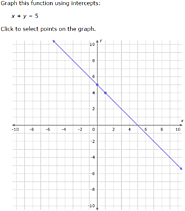Updated by Josie Linder on Dec 08, 2016
REPORT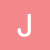Josie Linder
Owner
10 items   1 followers   0 votes   18 views

# Slope of a Graph

Everything you need to know about slope:

• finding slope from two points
• positive, negative, zero, undefined
• equations in slope intercept form
• parallel/perpendicular

fulfills standards: F-IF

1

## Slope DudeThis short video shows the adventure of Slope Dude, which uses the ski run of Slope Dude to demonstrate positive slope, negative slope, a slope of zero, and an undefined slope. This was the most valuable resource to me going into Algebra. Although it is silly, I still think back to this video when finding slope.

2

## Slope Music Video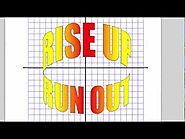Music Video about parts of a graph, slope, and slope-intercept form. This is a fun song that will likely get stuck in the kids' heads. It covers a variety of topics. YouTube is a popular resource and the video will link to like videos.

3

## Slopes of Parallel and Perpendicular Lines ( GMAT / GRE / CAT / Bank PO / SSC CGL)This video shows how to identify parallel and perpendicular lines with examples. This is a clear video. The girl has a cool accent which makes it interesting.

4

## Finding slope from graph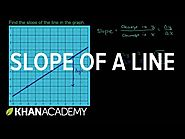The slope of a line is rise over run. Learn how to calculate the slope of the line in a graph by finding the change in y and the change in x. This is basic, fundamental solution to slope. Khan academy was my saving grace in high school. It is a great tool that students can come back to to learn more.

5

## Finding the Slope of a Line from Two Points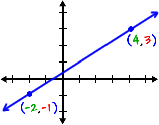Finding m (slope) when given two points. Nice graphics, easy to use. Many more pages about slope.

6

## Writing linear equations using the slope-intercept form (Algebra 1, Formulating linear equations) – MathplanetWhere m is the slope of the line and b is the y-intercept. You can use this equation to write an equation if you know the slope and the y-intercept. Clear step-by-step instructions

7

## Perpendicular and Parallel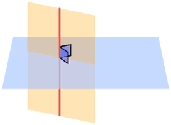This site mathematically defines parallel and perpendicular lines. Interactive - students can practice graphing. Thumbs up!

8

## Given two points, write y=mx+b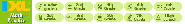Using two points on the same line, find the slope and the y-intercept. Then write the equation of the line in slope intercerpt form. Also, you may be asked to do only one step in this process (find m or find b). Game style, interactive questions.

9